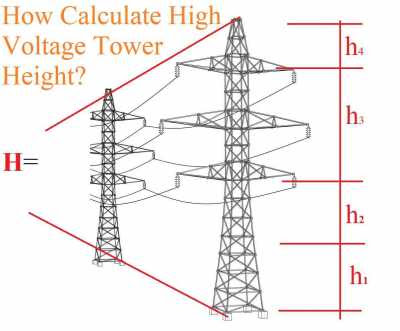WAZIPOINT Engineering Science & Technology: How to Calculate Transmission Line Tower Height?

##Height of Tower Structure for High Voltage Transmission Lines:

Towe height is the major factor to design an overhead transmission line tower structure. The tower design philosophy mainly consider the factors tower height, base width, top damper width and cross arm's length. Typical tower height ranges from 15 to 55 m (49 to 180 ft).

Height of tower is determined by-

H=h1+h2+h3+h4

where,
h=Minimum permissible ground clearance;
h=Maximum sag;
h=Vertical spacing between conductors;
h=Vertical clearance between earthwire and top conductor.

### Minimum Ground Clearance Calculation

The short cut method of calculation formula for minimum ground clearance is as-

CL=5.182+0.305*K

where,
K=(v-33)/33

As per Indian Electricity rules, 1956; the minimum permissible ground clearance is as below:

Permissible Ground Clearance:

Sl.NoVoltage LevelGround Clearance(m)Telecommunication Clearance(mm)Railway Clearance(m)
1<=33kV5.2024407.9/6.7
266kV5.4924407.9/6.7
3132kV6.1027408.5/7.3
4220kV7.0130508.8/7.6
5400kV8.84488011.2/10.0

### Maximum Sag and Tension calculation formula for overhead transmission lines conductor:

The sag in overhead transmission line conductor refers to the difference in level between the point of both supports and the lowest point on the conductor, simply the dip of the conductor between the two-level supports is called sag.

#### Factors affecting the sag

Conductor weight: Sag of the conductor is directly proportional to its weight. The weight of the conductors is increased due to ice loading.

Span: The Sag is directly proportional to the square of the span length. Longer span gives more sag.

Tension: The sag is inversely proportional to the tension in the conductor. Higher tension increases the stress in the insulators and supporting structures.

Wind: It increases sag in the inclined direction.
Temperature – The sag is reduced at low temperatures and is increases at higher temperatures.

We can use the following two formulas to calculate maximum sag and tension calculation, Parabolic formula for span length equal or less than 300m and Catenary formula for span length greater than 300m.

Parabolic formula:
L =S+(S3.q2)/24.H2
D =(S2.q)/8H
T/q =(S2.q)/8H +H/q
q =√(w2+p2)

Catenary formula:
L =(2H/q)Sinh (Sq/2H)
D =H/q[Cosh(Sq/2H)-1]
T/q =D+H/q
q =√(w2+p2)

Where,
q = resultant load on the conductor per unit length;
S = Span length;
D = Sag of the conductor at its lowest point;
L = Length of the conductor in span;
T = Tension at either point of support;
H = Horizontal tension at the lowest point.

### Vertical spacing between conductors

Conductor spacing or phase distance may calculate in different ways.

Mecom Formula:
Spacing (cm) = 0.3048*V+4.010(D/W)√S
wher,
V = System volltage in kV;
D = Diameter of conductor in cm;
S = Cable sagin cm;
W = Weight of conductor in Kg/m.

VDE Formula:
Spacing (cm) = 07.5√S + V2/2000
wher,
V = System volltage in kV;
S = Cable sagin cm.

Still formula:
Spacing (cm) = 5.08 + 1.814* V + [l/27.8]
wher,
l = Average span length(m)

NESC formula:
Spacing (cm) = 0.762*V + 3.681√S + L/√2
Where,
V= Voltage of system in KV;
S= Sag in cm;
L= Length of insulator string in cm.

Swedish formula:
Spacing (cm) = 6.5√S + 0.7*E
Where,
E= Line Voltage in KV;
S= Sag in cm.

French formula:
Spacing (cm) = 8.0√(S + L) + E/1.5
Where,
E= Line Voltage in KV;
S= Sag in cm;
L= length of insulating string(cm).

### Vertical clearance between earth wire and top conductor

Tower top clearance is the vertical clearance between earth wire and top conductor which is governed by the angle of shielding. The shield angle varies from about 250 to 300, depending on the configuration of conductors. Tower top clearance shall be taken 1.5 and 2.25 m for 132 kV and 220 kV respectively for 00 swings.

Tower height and strength may vary considering the line diversion or the line direction angle as below:

Type A Tower (Tangent Tower with suspension string)
Used on straight runs and up to 2° line diversion;
Type B Tower (Small Angle Tower with tension string)
Used for line deviation from 2° to 15°;
Type C Tower (Medium Angle Tower with tension string ).
Used for line deviation from 15° to 30°;

Type D Tower (Large angle tower with tension string)
Used for line deviation from 30° to 60°;

Type E Tower (Dead End Tower with tension string)
Used for line termination & starting;

Special tower-
Suspension Tower (Span ≈ 1000 m)
Used for River crossing, Mountain crossing etc.;
Transposition Tower
Used for transposition of the tower.

#### 1 comment:

1.IAS menyediakan layanan audit Sertifikasi ISO untuk berbagai standar internasional. Di luar dari Standar-Standar ini, sertifikat ISO 13485:2016 menetapkan persyaratan Sistem Manajemen Mutu perangkat / peralatan Medis yang secara konsisten memenuhi Pelanggan dan persyaratan peraturan yang berlaku. Sertifikasi ISO 13485. ias berfokus pada produsen untuk menghasilkan kepatuhan perangkat medis yang aman dan efektif terhadap standar 13485. +62 8111012541

WAZIPOINT:
Thank you very much to visit and valuable comments on this blog post. Keep in touch for next and new article. Share your friends and well-wisher, share your idea to worldwide.